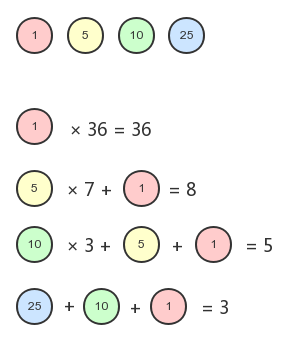# js贪心算法 钱币找零问题代码实例_咋地 _前端开发者

1. 如果全部用1美分的硬币，一共需要36个硬币
2. 如果用5美分的硬币，则需要7个5美分的硬币 + 1个1美分的硬币 = 8个硬币
3. 如果用10美分的硬币，则需要3个10美分的硬币 + 1个5美分的硬币 + 1个1美分的硬币 = 5个硬币
4. 如果用25美分的硬币，则需要1个25美分的硬币 + 1个10美分的硬币 + 1个1美分的硬币 = 3个硬币```function minCoinChange(coins, amount) {
let result = null;
if (!amount) return result;

const makeChange = (index, value, min) => {
let coin = coins[index];
let newAmount = Math.floor(value / coin);
if (newAmount) min[coin] = newAmount;
if (value % coin !== 0) {
makeChange(--index, value - coin * newAmount, min);
}
};

const arr = [];
for (let i = 0; i < coins.length; i++) {
const cache = {};
makeChange(i, amount, cache);
arr.push(cache);
}
console.log(arr);
let newMin = 0;
arr.forEach(item => {
let min = 0;
for (let v in item) min += item[v];
if (!newMin || min < newMin) {
newMin = min;
result = item;
}
});
return result;
}```

```const result = minCoinChange2([1, 5, 10, 25], 36);
console.log(result);```

```[
{ '1': 36 },
{ '1': 1, '5': 7 },
{ '1': 1, '5': 1, '10': 3 },
{ '1': 1, '10': 1, '25': 1 }
]
{ '1': 1, '10': 1, '25': 1 }```

» 本文链接地址：https://www.rokub.com/70113.html
» 您也可以订阅本站：https://www.rokub.com
64K

• 回顶